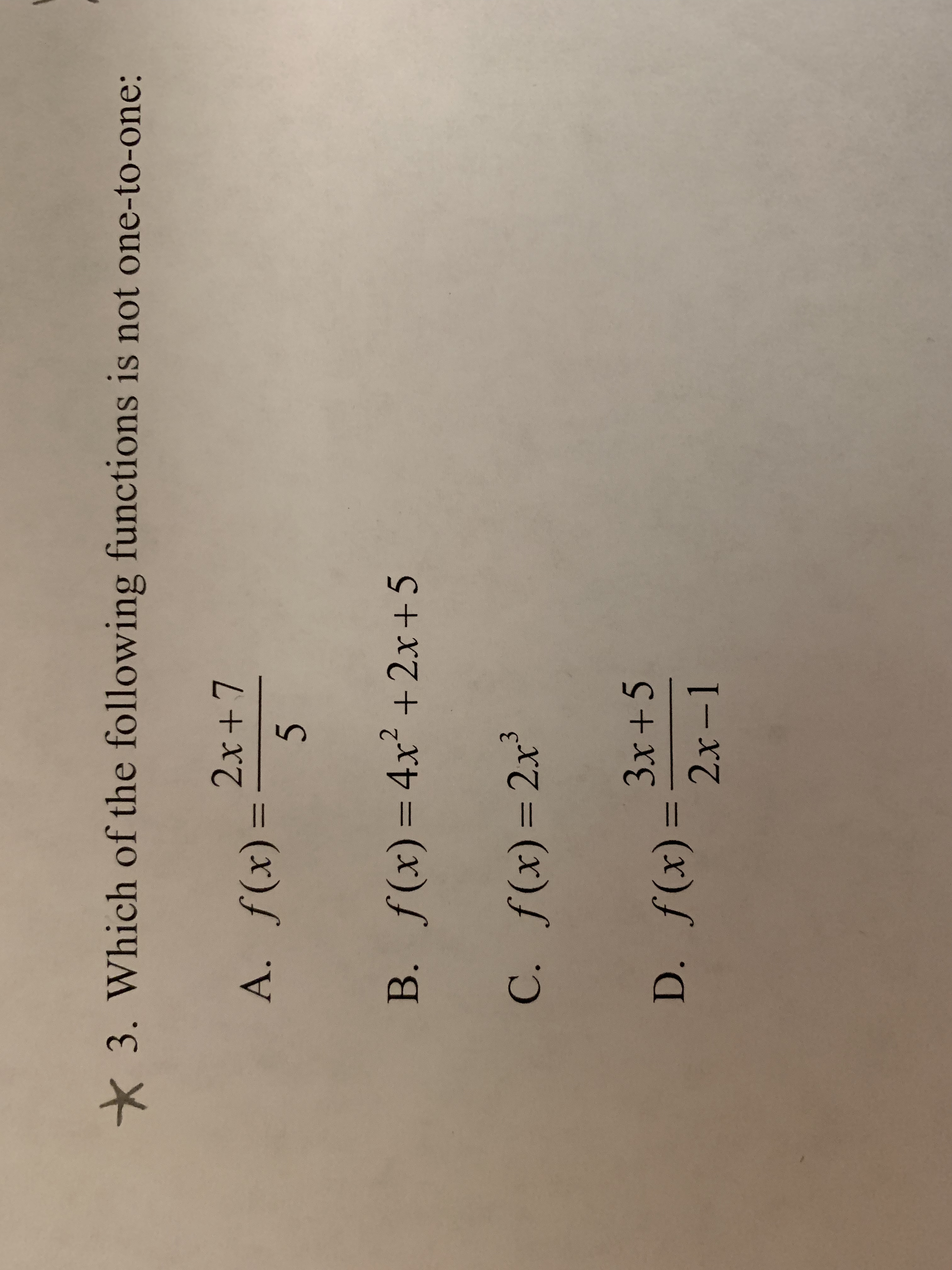3. Which of the following functions is not one-to-one22x +7f(x)=A,f(x)=4x2+2x + 5B.x)=2x3x +52x -11

Questionhelp_outlineImage Transcriptionclose3. Which of the following functions is not one-to-one 2 2x +7 f(x)= A, f(x)=4x2+2x + 5 B. x)=2x 3x +5 2x -1 1 fullscreen
Step 1

The definition of one-to-one function is stated as below.

Step 2

A.  Check whether the given function is one-to-one or not.

Step 3

B.  Check whether the given fu...

Want to see the full answer?

See Solution

Want to see this answer and more?

Our solutions are written by experts, many with advanced degrees, and available 24/7

See Solution
Tagged in

Other